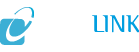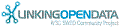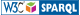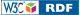#An Entity of Type : owl:Class, within Data Space : data.silknow.org associated with source document(s)

Scope note: This class comprises quantifiable properties that can be measured by some calibrated means and can be approximated by values, i.e. points or regions in a mathematical or conceptual space, such as natural or real numbers, RGB values etc. An instance of E54 Dimension represents the true quantity, independent from its numerical approximation, e.g. in inches or in cm. The properties of the class E54 Dimension allow for expressing the numerical approximation of the values of an instance of E54 Dimension. If the true values belong to a non-discrete space, such as spatial distances, it is recommended to record them as approximations by intervals or regions of indeterminacy enclosing the assumed true values. For instance, a length of 5 cm may be recorded as 4.5-5.5 cm, according to the precision of the respective observation. Note, that interoperability of values described in different units depends critically on the representation as value regions. Numerical approximations in archaic instances of E58 Measurement Unit used in historical records should be preserved. Equivalents corresponding to current knowledge should be recorded as additional instances of E54 Dimension as appropriate. Examples: - currency: £26.00 - length: 3.9-4.1 cm - diameter: 26 mm - weight: 150 lbs - density: 0.85 gm/cc - luminescence: 56 ISO lumens - tin content: 0.46 % - taille au garot: 5 hands - calibrated C14 date: 2460-2720 years, etc In First Order Logic: E54(x) ⊃ E1(x)

AttributesValues
rdf:type
rdfs:subClassOf
rdfs:label
• E54 Dimension (en)
rdfs:comment
• Scope note: This class comprises quantifiable properties that can be measured by some calibrated means and can be approximated by values, i.e. points or regions in a mathematical or conceptual space, such as natural or real numbers, RGB values etc. An instance of E54 Dimension represents the true quantity, independent from its numerical approximation, e.g. in inches or in cm. The properties of the class E54 Dimension allow for expressing the numerical approximation of the values of an instance of E54 Dimension. If the true values belong to a non-discrete space, such as spatial distances, it is recommended to record them as approximations by intervals or regions of indeterminacy enclosing the assumed true values. For instance, a length of 5 cm may be recorded as 4.5-5.5 cm, according to the precision of the respective observation. Note, that interoperability of values described in different units depends critically on the representation as value regions. Numerical approximations in archaic instances of E58 Measurement Unit used in historical records should be preserved. Equivalents corresponding to current knowledge should be recorded as additional instances of E54 Dimension as appropriate. Examples: - currency: £26.00 - length: 3.9-4.1 cm - diameter: 26 mm - weight: 150 lbs - density: 0.85 gm/cc - luminescence: 56 ISO lumens - tin content: 0.46 % - taille au garot: 5 hands - calibrated C14 date: 2460-2720 years, etc In First Order Logic: E54(x) ⊃ E1(x) (en)
notation
• E54
is rdf:type of
Faceted Search & Find service v1.16.103 as of Jun 21 2021OpenLink Virtuoso version 07.20.3232 as of Jun 21 2021, on Linux (x86_64-pc-linux-musl), Single-Server Edition (125 GB total memory)